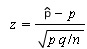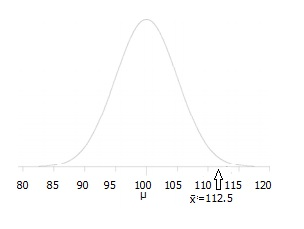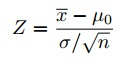# Hypothesis Testing

Share on

## Hypothesis TestingThe main purpose of statistics is to test a hypothesis. For example, you might run an experiment and find that a certain drug is effective at treating headaches. But if you can’t repeat that experiment, no one will take your results seriously. A good example of this was the cold fusion discovery, which petered into obscurity because no one was able to duplicate the results.

Watch the video for a brief overview of hypothesis testing:

Need help with a homework problem? Check out our tutoring page!

## What is a Hypothesis?

A hypothesis is an educated guess about something in the world around you. It should be testable, either by experiment or observation. For example:

• A new medicine you think might work.
• A way of teaching you think might be better.
• A possible location of new species.
• A fairer way to administer standardized tests.

It can really be anything at all as long as you can put it to the test.

## What is a Hypothesis Statement?

If you are going to propose a hypothesis, it’s customary to write a statement. Your statement will look like this:
“If I…(do this to an independent variable)….then (this will happen to the dependent variable).”
For example:

• If I (decrease the amount of water given to herbs) then (the herbs will increase in size).
• If I (give patients counseling in addition to medication) then (their overall depression scale will decrease).
• If I (give exams at noon instead of 7) then (student test scores will improve).
• If I (look in this certain location) then (I am more likely to find new species).

A good hypothesis statement should:

• Include an “if” and “then” statement (according to the University of California).
• Include both the independent and dependent variables.
• Be testable by experiment, survey or other scientifically sound technique.
• Be based on information in prior research (either yours or someone else’s).
• Have design criteria (for engineering or programming projects).

## What is Hypothesis Testing?Hypothesis testing in statistics is a way for you to test the results of a survey or experiment to see if you have meaningful results. You’re basically testing whether your results are valid by figuring out the odds that your results have happened by chance. If your results may have happened by chance, the experiment won’t be repeatable and so has little use.

Hypothesis testing can be one of the most confusing aspects for students, mostly because before you can even perform a test, you have to know what your null hypothesis is. Often, those tricky word problems that you are faced with can be difficult to decipher. But it’s easier than you think; all you need to do is:

1. Figure out your null hypothesis,
3. Choose what kind of test you need to perform,
4. Either support or reject the null hypothesis.

### What is the Null Hypothesis?

If you trace back the history of science, the null hypothesis is always the accepted fact. Simple examples of null hypotheses that are generally accepted as being true are:

1. DNA is shaped like a double helix.
2. There are 8 planets in the solar system (excluding Pluto).
3. Taking Vioxx can increase your risk of heart problems (a drug now taken off the market).

### How do I State the Null Hypothesis?

You won’t be required to actually perform a real experiment or survey in elementary statistics (or even disprove a fact like “Pluto is a planet”!), so you’ll be given word problems from real-life situations. You’ll need to figure out what your hypothesis is from the problem. This can be a little trickier than just figuring out what the accepted fact is. With word problems, you are looking to find a fact that is nullifiable (i.e. something you can reject).

## Hypothesis Testing Examples #1: Basic Example

A researcher thinks that if knee surgery patients go to physical therapy twice a week (instead of 3 times), their recovery period will be longer. Average recovery times for knee surgery patients is 8.2 weeks.

The hypothesis statement in this question is that the researcher believes the average recovery time is more than 8.2 weeks. It can be written in mathematical terms as:
H1: μ > 8.2

Next, you’ll need to state the null hypothesis (See: How to state the null hypothesis). That’s what will happen if the researcher is wrong. In the above example, if the researcher is wrong then the recovery time is less than or equal to 8.2 weeks. In math, that’s:
H0 μ ≤ 8.2

## Rejecting the null hypothesis

Ten or so years ago, we believed that there were 9 planets in the solar system. Pluto was demoted as a planet in 2006. The null hypothesis of “Pluto is a planet” was replaced by “Pluto is not a planet.” Of course, rejecting the null hypothesis isn’t always that easy—the hard part is usually figuring out what your null hypothesis is in the first place.

## Hypothesis Testing Examples (One Sample Z Test)

The one sample z test isn’t used very often (because we rarely know the actual population standard deviation). However, it’s a good idea to understand how it works as it’s one of the simplest tests you can perform in hypothesis testing. In English class you got to learn the basics (like grammar and spelling) before you could write a story; think of one sample z tests as the foundation for understanding more complex hypothesis testing. This page contains two hypothesis testing examples for one sample z-tests.

## One Sample Hypothesis Testing Example: One Tailed Z Test

Watch the video for an example:

A principal at a certain school claims that the students in his school are above average intelligence. A random sample of thirty students IQ scores have a mean score of 112.5. Is there sufficient evidence to support the principal’s claim? The mean population IQ is 100 with a standard deviation of 15.

Step 1: State the Null hypothesis. The accepted fact is that the population mean is 100, so: H0: μ = 100.

Step 2: State the Alternate Hypothesis. The claim is that the students have above average IQ scores, so:
H1: μ > 100.
The fact that we are looking for scores “greater than” a certain point means that this is a one-tailed test.Step 4: State the alpha level. If you aren’t given an alpha level, use 5% (0.05).

Step 5: Find the rejection region area (given by your alpha level above) from the z-table. An area of .05 is equal to a z-score of 1.645.

Step 6: Find the test statistic using this formula:For this set of data: z= (112.5 – 100) / (15/√30) = 4.56

Step 6: If Step 6 is greater than Step 5, reject the null hypothesis. If it’s less than Step 5, you cannot reject the null hypothesis. In this case, it is more (4.56 > 1.645), so you can reject the null.

## One Sample Hypothesis Testing Examples: #3

Watch the video for an example of a two-tailed z-test:

Blood glucose levels for obese patients have a mean of 100 with a standard deviation of 15. A researcher thinks that a diet high in raw cornstarch will have a positive or negative effect on blood glucose levels. A sample of 30 patients who have tried the raw cornstarch diet have a mean glucose level of 140. Test the hypothesis that the raw cornstarch had an effect.

Step 1: State the null hypothesis: H0:μ=100
Step 2: State the alternate hypothesis: H1:≠100
Step 3: State your alpha level. We’ll use 0.05 for this example. As this is a two-tailed test, split the alpha into two.
0.05/2=0.025
Step 4: Find the z-score associated with your alpha level. You’re looking for the area in one tail only. A z-score for 0.75(1-0.025=0.975) is 1.96. As this is a two-tailed test, you would also be considering the left tail (z = 1.96)
Step 5: Find the test statistic using this formula:z = (140 – 100) / (15/√30) = 14.60.
Step 6: If Step 5 is less than -1.96 or greater than 1.96 (Step 3), reject the null hypothesis. In this case, it is greater, so you can reject the null.

*This process is made much easier if you use a TI-83 or Excel to calculate the z-score (the “critical value”).
See:

## Hypothesis Testing Examples: Mean (Using TI 83)

You can use the TI 83 calculator for hypothesis testing, but the calculator won’t figure out the null and alternate hypotheses; that’s up to you to read the question and input it into the calculator.

Example problem: A sample of 200 people has a mean age of 21 with a population standard deviation (σ) of 5. Test the hypothesis that the population mean is 18.9 at α = 0.05.

Step 1: State the null hypothesis. In this case, the null hypothesis is that the population mean is 18.9, so we write:
H0: μ = 18.9

Step 2: State the alternative hypothesis. We want to know if our sample, which has a mean of 21 instead of 18.9, really is different from the population, therefore our alternate hypothesis:
H1: μ ≠ 18.9

Step 3: Press Stat then press the right arrow twice to select TESTS.

Step 4: Press 1 to select 1:Z-Test…. Press ENTER.

Step 5: Use the right arrow to select Stats.

Step 6: Enter the data from the problem:
μ0: 18.9
σ: 5
x: 21
n: 200
μ: ≠μ0

Step 7: Arrow down to Calculate and press ENTER. The calculator shows the p-value:
p = 2.87 × 10-9

This is smaller than our alpha value of .05. That means we should reject the null hypothesis.

## Bayesian Hypothesis Testing: What is it?

Bayesian hypothesis testing helps to answer the question: Can the results from a test or survey be repeated?
Why do we care if a test can be repeated? Let’s say twenty people in the same village came down with leukemia. A group of researchers find that cell-phone towers are to blame. However, a second study found that cell-phone towers had nothing to do with the cancer cluster in the village. In fact, they found that the cancers were completely random. If that sounds impossible, it actually can happen! Clusters of cancer can happen simply by chance. There could be many reasons why the first study was faulty. One of the main reasons could be that they just didn’t take into account that sometimes things happen randomly and we just don’t know why.

## P Values.

It’s good science to let people know if your study results are solid, or if they could have happened by chance. The usual way of doing this is to test your results with a p-value. A p value is a number that you get by running a hypothesis test on your data. A P value of 0.05 (5%) or less is usually enough to claim that your results are repeatable. However, there’s another way to test the validity of your results: Bayesian Hypothesis testing. This type of testing gives you another way to test the strength of your results.

## Bayesian Hypothesis Testing.

Traditional testing (the type you probably came across in elementary stats or AP stats) is called Non-Bayesian. It is how often an outcome happens over repeated runs of the experiment. It’s an objective view of whether an experiment is repeatable.
Bayesian hypothesis testing is a subjective view of the same thing. It takes into account how much faith you have in your results. In other words, would you wager money on the outcome of your experiment?

## Differences Between Traditional and Bayesian Hypothesis Testing.

Traditional testing (Non Bayesian) requires you to repeat sampling over and over, while Bayesian testing does not. The main different between the two is in the first step of testing: stating a probability model. In Bayesian testing you add prior knowledge to this step. It also requires use of a posterior probability, which is the conditional probability given to a random event after all the evidence is considered.

## Arguments for Bayesian Testing.

Many researchers think that it is a better alternative to traditional testing, because it:

1. Includes prior knowledge about the data.
2. Takes into account personal beliefs about the results.

## Arguments against.

1. Including prior data or knowledge isn’t justifiable.
2. It is difficult to calculate compared to non-Bayesian testing.

## Hypothesis Testing Articles

Basics:

Specific Tests:

Related Articles:

## References

Gonick, L. and Smith, W. The Cartoon Guide to Statistics. New York: Harper Perennial, pp. 140-142, 1993.
Good, P. Permutation Tests: A Practical Guide to Resampling Methods for Testing Hypotheses, 2nd ed. New York: Springer-Verlag, 2000.
Hoel, P. G.; Port, S. C.; and Stone, C. J. “Testing Hypotheses.” Ch. 3 in Introduction to Statistical Theory. New York: Houghton Mifflin, pp. 52-110, 1971.

CITE THIS AS:
Stephanie Glen. "Hypothesis Testing" From StatisticsHowTo.com: Elementary Statistics for the rest of us! https://www.statisticshowto.com/probability-and-statistics/hypothesis-testing/
---------------------------------------------------------------------------Need help with a homework or test question? With Chegg Study, you can get step-by-step solutions to your questions from an expert in the field. Your first 30 minutes with a Chegg tutor is free!